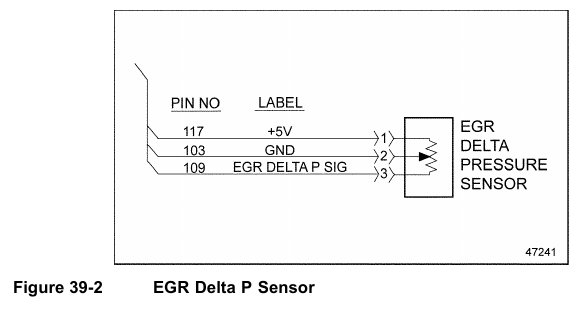# Series 60 – SPN 411/FMI 4 – EGR DIFFERENTIAL PRESSURE OR DELTA P SENSOR CIRCUIT OUTSIDE OF NORMAL OPERATING RANGE

This diagnosis is typically EGR Delta P Sensor short to ground.

39.3.1
Short to Ground Check
Check as follows:
1. Disconnect the EGR Delta P Sensor.
2. Disconnect the 120–pin MCM connector.
3. Measure the resistance between pins 1 and 2 of the EGR Delta P Sensor.
[a] If the resistance is greater than 5Ω, go to step 4.
[b] If the resistance is less than 5Ω, repair the short between pins 103 and 117 of the
120–pin MCM connector. Refer to section 39.3.1.1.
4. Measure the resistance between pins 2 and 3 of the EGR Delta P Sensor.
[a] If the resistance is greater than 5Ω, go to step 5.
[b] If the resistance is less than 5Ω, repair the short between pins 103 and 109 of the
120–pin MCM connector. See Figure 39-2. Refer to section 39.3.1.1.5. Measure the resistance between pin 1 of the EGR Delta P Sensor and ground.
[a] If the resistance is greater than 3Ω, go to step 6.
[b] If the resistance is less than 3Ω, repair the short circuit between pin 1 of the EGR
Delta P Sensor harness connector and ground. Refer to section 39.3.1.1.
6. Measure the resistance between pin 3 of the EGR Delta P Sensor and ground.
[a] If the resistance is greater than 3Ω, repeat steps 3 through 6. If the results are the
same, call the Detroit Diesel Customer Support Center (313–592–5800).
[b] If the resistance is less than 3Ω, repair the short circuit between pin 3 of the EGR
Delta P Sensor harness connector and ground. Refer to section 39.3.1.1.

39.3.1.1
Verify Repairs
Verify repairs as follows:
1. Turn ignition OFF.
2. Reconnect any electrical connections that were disconnected to perform the diagnosis.